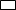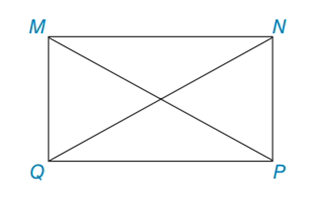Chapter 4.3, Problem 12EElementary Geometry For College St...

7th Edition
Alexander + 2 others
ISBN: 9781337614085

Solutions

Chapter
SectionElementary Geometry For College St...

7th Edition
Alexander + 2 others
ISBN: 9781337614085
Textbook ProblemIn Exercises 11 to 14, consider MNPQ with diagonals M P ¯  and  N Q ¯ . When the answer is not a whole number, leave a square root answer.Exercises 11 – 14If QP = 9 and NP = 6, find NQ and MP.

To determine

To find:

NQ and MP of the given rectangle.

Explanation

The basic property of rectangle:

1) All the properties of a parallelogram apply (the ones that matter here are parallel sides, opposite sides are congruent, and diagonals bisect each other).

2) All angles are right angles by definition.

3) The diagonals are congruent.

Calculation:

Given: QP = 9 and NP = 6

Consider the rectangle.

To find NQ and MP:

From the property, opposite sides are congruent in rectangle.

So, MN = QP = 9

MQ = NP = 6

Let us take right MPQ.

By using Pythagorean Theorem

MP2=QP2+QM2=92+62=81+36=117MP=117

Let us take right QNP

Still sussing out bartleby?

Check out a sample textbook solution.

See a sample solution

The Solution to Your Study Problems

Bartleby provides explanations to thousands of textbook problems written by our experts, many with advanced degrees!

Get Started

Simplify the expressions in Exercises 97106. x1/2y2x1/2y

Finite Mathematics and Applied Calculus (MindTap Course List)

In words, explain what is measured by SS, variance, and standard deviation.

Statistics for The Behavioral Sciences (MindTap Course List)

Write the following numbers in word form. 3. .081

Contemporary Mathematics for Business & Consumers

Evaluate 6 8 24 30

Study Guide for Stewart's Multivariable Calculus, 8th

By definition the improper integral It is not an improper integral.

Study Guide for Stewart's Single Variable Calculus: Early Transcendentals, 8th